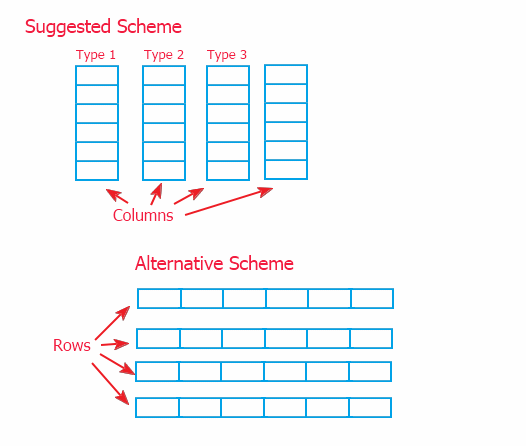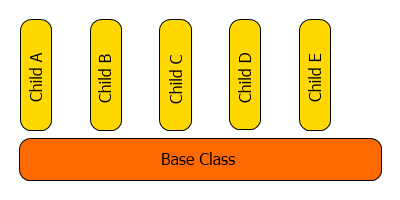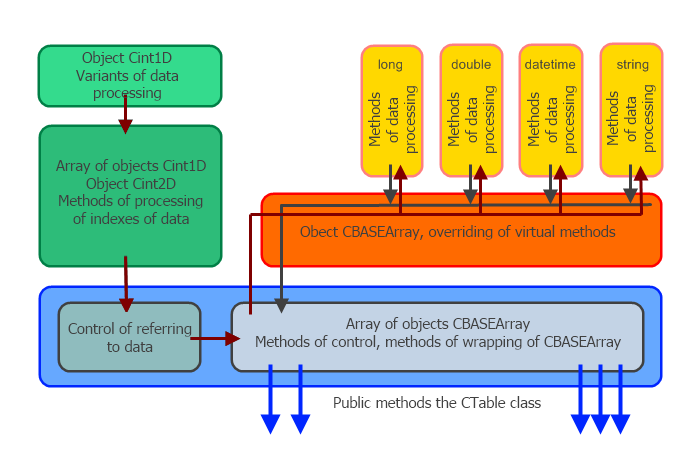# 外汇EA编写教程：MQL5中的电子表格

### 1。分类方法说明

1.1。首次调整大小

`void  FirstResize(const ENUM_DATATYPE &TYPE[]);`

`void  SecondResize(int j);`

1.3。第一尺寸

```int   FirstSize();
```

`int   SecondSize();`

1.5。修剪台

```void   PruningTable(int count);
```

1.6。可复制的

`void   CopyTable(CTable *sor);`

`void   CopyTable(CTable *sor,int sec_beg,int sec_end);`

1.7。可打印的

`ENUM_DATATYPE  TypeTable(int i)`

1.8。变化

change（）方法执行列交换。

`bool   Change(int &sor0,int &sor1);`

1.9。插入

`bool   Insert(int rec,int sor);`

1.10。变体/变体副本

```void   variant(int ind);
int    variant();
void   variantcopy(int rec,int sor);```

variant（int ind）方法切换所选变量。执行自动内存分配。如果指定的参数数目小于上次指定的参数数目，则不会重新分配内存。

variantcopy方法允许将“sor”变量复制到“rec”变量。创建此函数是为了排列变量。如果“rec”变量不存在，它会自动增加变量的数量并切换到新复制的变量。

1.11。SortTwodim数组

SortTwodiMaray方法按所选行“i”对表进行排序。

`void   SortTwoDimArray(int i,int beg,int end,bool mode=false);`

```int   QuickSearch(int i,long element,int beg,int end,bool mode=false);
```

1.13。搜索第一个

```int  SearchFirst(int i,long element,int beg,int end,bool mode=false);
```

1.14。搜索上次

```int   SearchLast(int i,long element,int beg,int end,bool mode=false);
```

1.15。搜索大

`int   SearchGreat(int i,long element,int beg,int end,bool mode=false);`

1.16。无搜索

```int  SearchLess(int i,long element,int beg,int end,bool mode=false);
```

set和get函数有空类型；它们由表中使用的四种数据类型覆盖。此函数标识数据类型，如果“value”参数与列类型不匹配，将显示一条警告消息，并且不会分配。唯一的例外是字符串类型。如果输入参数是字符串类型，它将转换为列类型。当无法设置接收单元值的变量时，此异常被定制以便于信息的传输。

```void   Set(int i,int j,long     value); // setting value of the i-th row and j-th column
void   Set(int i,int j,double   value); // setting value of the i-th row and j-th columns
void   Set(int i,int j,datetime value);// setting value of the i-th row and j-tj column
void   Set(int i,int j,string   value); // setting value of the i-th row and j-th column
```

```   //--- getting value
void   Get(int i,int j,long     &recipient); // getting value of the i-th row and j-th column
void   Get(int i,int j,double   &recipient); // getting value of the i-th row and j-th column
void   Get(int i,int j,datetime &recipient); // getting value of the i-th row and j-th column
void   Get(int i,int j,string   &recipient); // getting value of the i-th row and j-th column
```

1.19。SGET

`string sGet(int i,int j); // return value of the i-th row and j-th column `

get序列的唯一函数通过“返回”运算符而不是参数变量返回值。返回字符串类型的值（不考虑列类型）。

```void  StringDigits(int i,int digits);
```

`int   StringDigits(int i);`

1.21。使用实例：

```#include <Table.mqh>
ENUM_DATATYPE TYPE=
{TYPE_LONG,TYPE_LONG,TYPE_STRING,TYPE_DATETIME,TYPE_STRING,TYPE_STRING,TYPE_DOUBLE};
//     0          1          2            3              4            5            6   //7
void OnStart()
{
CTable table,table1;
table.FirstResize(TYPE);             // dividing table, determining column types
table.SecondResize(5);               // change the number of rows

table.Set(6,0,"321.012324568");        // assigning data to the 6-th column, 0 row
table.Insert(2,6);                   // insert 6-th column in the 2-nd position
table.PruningTable(3);               // cut the table to 3 columns
table.StringDigits(2,5);             // set precision of 5 digits after the decimal point
Print("table ",table.sGet(2,0));       // print the cell located in the 2-nd column, 0 row

table1.CopyTable(GetPointer(table));  // copy the entire table 'table' to the 'table1' table
table1.StringDigits(2,8);            // set 8-digit precision
Print("table1 ",table1.sGet(2,0));     // print the cell located in the 2-nd column, 0 row of the 'table1' table.
}```

```2011.02.09 14:18:37     Table Script (EURUSD,H1)        table1 321.01232000
2011.02.09 14:18:37     Table Script (EURUSD,H1)        table 321.01232```

### 2。选择模型### 3。动态阵列中的统一数据

```class CBase
{
public:
CBase(){Print(__FUNCTION__);};
~CBase(){Print(__FUNCTION__);};
virtual void       set(int sor){};
virtual void       set(double sor){};
virtual int        get(int k){return(0);};
virtual double     get(double k){return(0);};
};
//+------------------------------------------------------------------+
class CA: public CBase
{
private:
int              temp;
public:
CA(){Print(__FUNCTION__);};
~CA(){Print(__FUNCTION__);};
void              set(int sor){temp=sor;};
int               get(int k){return(temp);};
};
//+------------------------------------------------------------------+
class CB: public CBase
{
private:
double            temp;
public:
CB(){Print(__FUNCTION__);};
~CB(){Print(__FUNCTION__);};
void             set(double sor){temp=sor;};
double           get(double k){return(temp);};
};
//+------------------------------------------------------------------+
void OnStart()
{
CBase *a;   CBase *b;
a=new CA(); b=new CB();
a.set(15);  b.set(13.3);
Print("a=",a.get(0)," b=",b.get(0.));
delete a;
delete b;
}
```### 4。操作阵列

```before sorting by the 2-nd column
4 2 3
1 5 3
3 3 6
after sorting
1 5 3
3 3 6
4 2 3
Initial array looks as following:
a= 4; a= 2; a= 3;
a= 1; a= 5; a= 3;
a= 3; a= 3; a= 6;
And the array of indexes of sorting by the 2-nd column looks as:
r=1;
r=2;
r=0;
Sorted values are returned according to the following scheme:
a[r]-> 1; a[r]-> 5; a[r]-> 3;
a[r]-> 3; a[r]-> 3; a[r]-> 6;
a[r]-> 4; a[r]-> 2; a[r]-> 3;```

### 5。二维阵列布置

```class CarrayInt
{
public:
~CarrayInt(){};
int               array[];
};
//+------------------------------------------------------------------+
class CTwoarrayInt
{
public:
~CTwoarrayInt(){};
CarrayInt         array[];
};
//+------------------------------------------------------------------+
void OnStart()
{
CTwoarrayInt two;
two.array.array;
}```

### 6。程序结构

CCTLE类的代码是使用本文中介绍的模板编写的（使用伪模板而不是C++模板）。正是因为模板的使用，我才能够如此快速地编写如此庞大的代码。这就是为什么我不打算详细说明整个代码；而且，算法的大多数代码都是对标准类的修改。CTable类还执行数据处理方法（在CTable类的实例中）和控制CINT2D类索引的对象之间的协调。整个协调包装采用的是通用的覆盖方式。

CTabable类中经常重复的覆盖率被定义替换，以避免生成过长的代码：

```#define _CHECK0_ Print(__FUNCTION__+"("+(string)i+","+(string)j+")");return;
#define _CHECK_ Print(__FUNCTION__+"("+(string)i+")");return(-1);
#define _FIRST_ first_data[aic[i]]
#define _PARAM0_ array_index.Ind(j),value
#define _PARAM1_ array_index.Ind(j),recipient
#define _PARAM2_ element,beg,end,array_index,mode```

`int QuickSearch(int i,long element,int beg,int end,bool mode=false){if(!check_type(i,TYPE_LONG)){_CHECK_}return(_FIRST_.QuickSearch(_PARAM2_));};`

`int QuickSearch(int i,long element,int beg,int end,bool mode=false){if(!check_type(i,TYPE_LONG)){Print(__FUNCTION__+"("+(string)i+")");return(-1);} return(first_data[aic[i]].QuickSearch(element,beg,end,array_index,mode));};`

`void CLONGArray::QuickSort(long &m_data[],Cint2D &index,int beg,int end,bool mode=0)`

```...
if(i<=j)
{
t=m_data[i];            it=index.Ind(i);
m_data[i++]=m_data[j];  index.Ind(i-1,index.Ind(j));
m_data[j]=t;            index.Ind(j,it);
if(j==0) break;
else     j--;
}
...```

### 总结

，网址为https://www.mql5.com/ru/articles/228。

MyFxtop迈投(www.myfxtop.com)-靠谱的外汇跟单社区，免费跟随高手做交易！